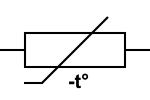# NTC Thermistor Probe

## What is a NTC (EN) - CTN (FR) thermistor probe?

NTC stands for "negative temperature coefficient", called CTN in French "negative temperature coefficient". NTC thermistors are resistors with a negative temperature coefficient, which means that resistance decreases as temperature increases. They are mainly used as resistive temperature sensors and current limiting devices. The coefficient of sensitivity to temperature is about five times that of silicon temperature sensors (silistors) and about ten times that of resistance temperature detectors (RTDs). NTC sensors are generally used in a range of -55 ° C to 200 ° C.

Definition: An NTC thermistor is a thermosensitive resistance whose resistance presents a significant, precise and predictable decrease as the central temperature of the resistance increases in the operating temperature range.

There are 3 products.

Sort by:

Active filters

### NTC 10k glove finger probe - G1 / 2 "connection - Plunger ...

• G1 / 2 "connection
• -40 To 105 ° C
• NTC 10K
• Custom cable
Price 10,89 €
227 products In stock

### Standard waterproof 10kOhm NTC probe

• 5 mm diameter
• -40 to 105 ° C
• NTC 10K
• Custom cable
Price 3,57 €
2063 products In stock

## Characteristics of NTC - CTN thermistors

Unlike resistance temperature detectors, which are made from metals, NTC thermistors are generally made of ceramics or polymers. Different materials used result in different temperature responses, as well as other characteristics.

## T ° / R curves of NTC - CTN thermistor probes

The temperature sensitivity of an NTC sensor is expressed in "percentage change per degree ° C". Depending on the materials used and the specifics of the production process, typical values ​​for temperature sensitivities range from -3% to -6% per ° CWhile most NTC thermistors are generally suitable for use in a temperature range of -55 ° C to 200 ° C, when they give their most accurate readings, there are special families of NTC thermistors that can be used at temperatures close to absolute zero (-273,15 ° C) as well as those specially designed for use above 150 ° C.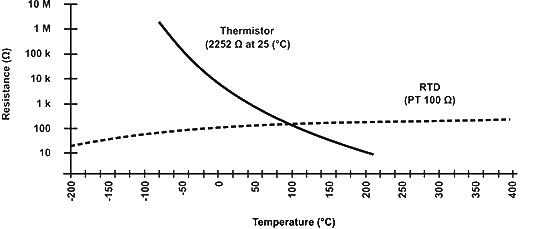As can be seen in the figure, NTC thermistors have a much steeper resistance-temperature slope than platinum alloy RTDs, which results in better temperature sensitivity. Nevertheless, RTD sensors (PLATINUM RESISTANCE THERMOMETERS type Pt100) remain the most precise sensors with an accuracy of ± 0,5% of the measured temperature and they are useful in the temperature range between -200 ° C and 800 ° C, a much wider range than that of NTC temperature sensors.

## Comparison with other temperature sensors

Compared to RTD, the NTC they have a smaller size, faster response, greater impact and vibration resistance at lower cost. They are slightly less precise than RTDs. Compared to thermocouples, the accuracy obtained from both is similar; however, thermocouples can withstand very high temperatures (up to over 1000 ° C) and are used in such applications instead of NTC - CTN thermistors, where they are sometimes referred to as pyrometers. Despite this, the thermistors NTC offers higher sensitivity, stability and accuracy than thermocouples at lower temperatures and are used with fewer additional circuits and therefore at a lower total cost. The cost is further reduced by the lack of need for signal conditioning circuits (amplifiers, level converters, etc.) often needed for RTDs and still needed for thermocouples.

#### Risk of self-heating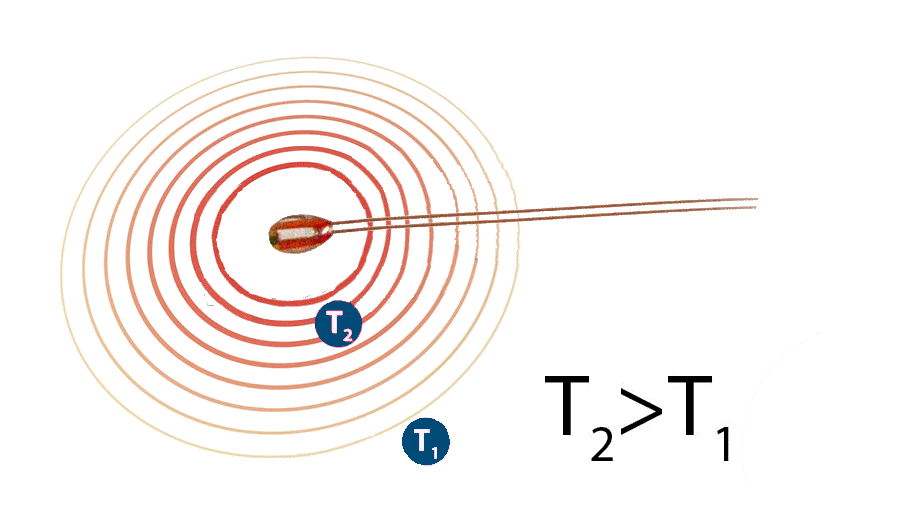The self-heating effect is a phenomenon that occurs every time a current flows through the NTC thermistor. Since the thermistor is essentially a resistance, it dissipates energy in the form of heat when there is current flowing through it. This heat is generated in the center of the thermistor and affects the accuracy of the measurements.. The extent to which this occurs depends on the amount of current flowing, the environment (whether it is a liquid or a gas, whether there is a flow on the NTC sensor, etc. .), the temperature coefficient of the thermistor and the condition of the thermistor. The fact that the resistance of the NTC sensor and therefore the current flowing through it depends on the environment is often used in detectors for the presence of liquids such as those found in storage tanks.

#### Thermal capacity

The thermal capacity represents the quantity of heat necessary to increase the temperature of the thermistor of 1 ° C and is usually expressed in mJ / ° C. Knowing the precise heat capacity is of great importance when using an NTC thermistor sensor as an inrush current limiting device, as it defines the response speed of the NTC temperature sensor.

#### Selection and calculation of the curve

The rigorous selection process must take into account the dissipation constant of the thermistor, the thermal time constant, the resistance value, the resistance-temperature curve and tolerances, to mention the most important factors.

Since the relationship between resistance and temperature (the RT curve) is highly non-linear, certain approximations must be used in practical system designs.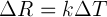First order approximation: When k is the negative temperature coefficient, ΔT is the temperature difference and ΔR is the change in resistance resulting from the change in temperature. This first order approximation is only valid for a very narrow temperature range and can only be used for temperatures where k is almost constant over the entire temperature range.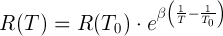Beta formula: Another equation gives satisfactory results, with an accuracy of ± 1 ° C over the range of 0 ° C to + 100 ° C. It depends on a single material constant β obtainable by measurements. The equation can be written as follows:

Where R (T) is the temperature resistance T in Kelvin, R (T 0 ) is a reference point at temperature T 0 . The beta formula requires a two-point calibration, which is generally not more accurate than ± 5 ° C over the entire useful range of the NTC thermistor.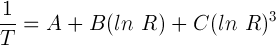Steinhart-Hart equation: The best known approximation to date is the Steinhart-Hart formula, published in 1968. Where ln R is the natural logarithm of the temperature resistance T in Kelvin, and A, B and C are coefficients derived from experimental measurements. These coefficients are generally published by the thermistor suppliers in the technical sheet. The Steinhart-Hart formula is generally accurate to approximately ± 0,15 ° C over the range of -50 ° C to + 150 ° C, which is sufficient for most applications. If higher accuracy is required, the temperature range should be reduced and accuracy better than ± 0,01 ° C over the range of 0 ° C to + 100 ° C is possible.

## Choose the correct approximation

The choice of formula used to calculate the temperature from the resistance measurement should be based on the available computing power, as well as the actual tolerance requirements. In some applications, a first-order approximation is more than enough, while in others, even the Steinhart-Hart equation does not meet the requirements and the thermistor must be calibrated point by point, performing a large number of measurements. and creating a correspondence table.

####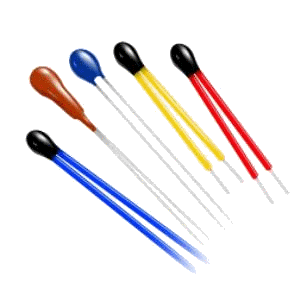Epoxy encapsulated thermistors

These NTC thermistors are made from metal oxides (manganese, cobalt, copper and nickel) frittés in the ceramic body. They generally offer quick response times, better stability and allow operation at higher temperatures than Disk and Chip NTC sensors, but they are more fragile. It is common to seal them in glass, to protect them against mechanical damage during assembly and to improve their measurement stability. Typical sizes range from 0,075 to 5mm in diameter.

####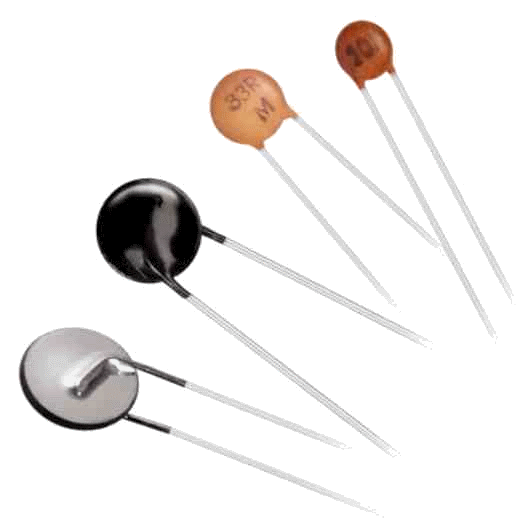Disc and chip thermistors

These NTC thermistors have metallized surface contacts. They are larger and have slower reaction times than NTC ball type resistors. However, due to their size, they have a higher dissipation constant (power required to raise their temperature by 1 ° C) and, since the puIssance dissipated by the thermistor is proportional to the square of the current, they support higher currents like thermistors. Disc thermistors are made by pressing a mixture of oxide powders into a round die, which is then sintered at high temperature. Chips are generally made by a ribbon molding process where a slurry of material is spread in the form of a thick film, dried and cut. Typical sizes range from 0,25 to 25mm in diameter.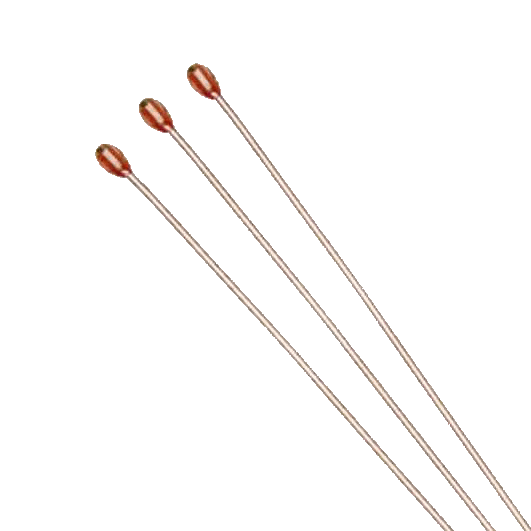#### NTC thermistors encapsulated in glass

These are NTC temperature sensors sealed in an airtight glass bubble. They are designed to be used with temperatures above 150 ° C or for mounting on a printed circuit, where robustness is essential. Encapsulate a thermistor ins the glass improves the stability of the sensor and protects the sensor from the environment. They are made by hermetically sealing NTC pearl-type resistors in a glass container. Typical sizes range from 0,4 to 10mm in diameter.

## NTC thermistor symbol

The following symbol is used for a thermistor with a negative temperature coefficient, in accordance with the IEC standard.